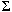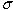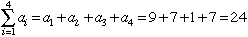Subject: Mathematics symbols What does the Sigma symbol mean in mathematics? Hi, Mathematicians use the same letter to stand for different concepts, depending on the situation. I will tell you two places where sigma is used but let me know if they are not what you are looking for. Sigma is a Greek letter that corresponds somewhat to our letter S and thus sigma is often used to stand for some concept that starts with S. There is an upper case (capital) sigma and a lower case (small) sigma. They areand.is most ofter used to indicate a sum. If you have a list of numbers, say a1=9, a2=7, a3=1 and a4=7 thenis used in statistics to mean "standard deviation". This is a measure of the spread or variation in a collection of numbers. For example if the 30 students in your class write a test and everyone gets between 70 and 80 then the standard deviation of the 30 scores would be quite small. If, however, the 30 scores range form the 40's to the 90's then the standard deviation would be larger. I hope this helps, Penny Go to Math Central